# Precision vs. Recall: Differences, Use Cases & Evaluation

Precision and recall are two measures of a machine learning model's performance. Learn about the difference between them and how to use them effectively.13
September 27, 2022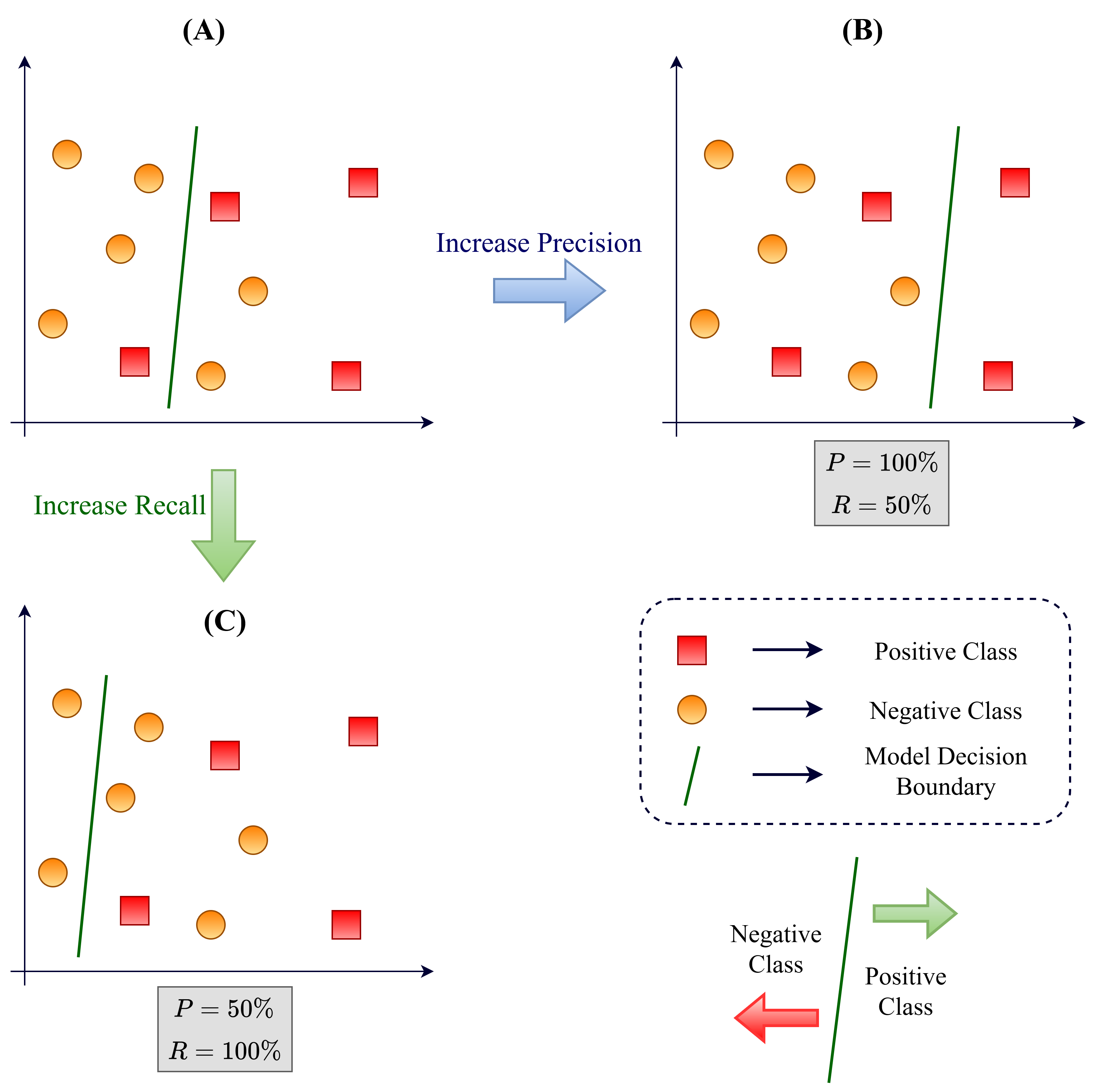Contents

The success or failure of machine learning models depends on how we evaluate their performance. Most of the time, the go-to metric for a coarse evaluation is model accuracy. However, it is neither comprehensive nor detailed.

Accuracy tells us how many times the model made correct predictions in the entire dataset. It does not give us any class-specific information like which class boundaries were learned well, where the model was more confused, etc.

Plus, in almost all real-world problems, the dataset is class imbalanced—different classes have different numbers of samples. In such cases, global accuracy is not a reliable indicator of the model’s quality. The model might get wrong predictions most of the time on the minority class (i.e., the class with a smaller number of samples), but the accuracy of the entire model might be high.

Precision and recall offer more insight into the model’s skill by elaborating its class-wise performance. These metrics are used differently based on the problem requirement—sometimes evaluating the precision is wiser, and sometimes recall is more important.

Global versions of the precision and recall metrics can also be calculated (using different strategies) to evaluate the overall performance of the model (like accuracy does).

Solve any video or image labeling task 10x faster and with 10x less manual work.

Explore other resources:

## Precision and Recall in Machine Learning

Precision is defined as the proportion of the positive class predictions that were actually correct. In other words, if a model classified a total of 100 samples to be of positive class, and 70 of them actually belonged to the positive class of the dataset (and 30 were negative class samples predicted incorrectly as “positive” by the classifier), then the precision is 70%.

Recall is defined as the proportion of actual positive class samples that were identified by the model. That is if the test set of a dataset consists of 100 samples in its positive class, how many of them were identified? If 60 of the positive samples were identified correctly, then the recall is 60%.

Multi-class classification problems can also be dissolved into one-vs-all binary problems—as discussed in our guide to the confusion matrix. Thus this concept of true positives, false positives, and false negatives still holds true. Precision and recall in such cases are calculated class-wise, giving us an idea of the decision boundaries learned by the model between each class of data.

But why is it important to know these class-wise metrics?

They help us identify the localized areas in the decision space where the model needs to improve. If a certain class out of a range of classes has poor performance, we can take corrective measures to see what the model is doing wrong there. Such class-wise metrics may also help identify problems in the underlying distribution of the data.

Maybe the quality of data belonging to this poorly performing class is not on par with the other classes because of problems like low-resolution of images, blurry image samples, or other kinds of noise that may hinder model performance due to inconsistency. Alternatively, maybe the expected labels of the samples in this class are not inherently correct, in which case the samples might need to be re-labeled by expert annotators in the domain.

## How to Calculate Precision and Recall?

To understand the precision and recall metrics, it is helpful to visualize them with the help of confusion matrices. A confusion matrix is an orderly representation of the predictive performance of a classifier on a dataset and can be used to calculate many different evaluation metrics (including accuracy, precision, and recall).

### Precision and Recall for Binary-Class Datasets

Let’s look at the precision and recall metrics from a mathematical standpoint with the help of confusion matrices. For a binary-class dataset, let’s call the classes “positive” and “negative” for the sake of simplicity.

Here are the essential definitions following this convention:

• True Positives (TP) are the number of positive class samples correctly classified by a model.
• True Negatives (TN) are the number of negative class samples correctly classified by a model.
• False Positives (FP) are the number of negative class samples that were predicted (incorrectly) to be of the positive class by the model.
• False Negatives (FN) are the number of positive class samples that were predicted (incorrectly) to be of the negative class by the model.

The confusion matrix for a binary-class dataset looks as shown below.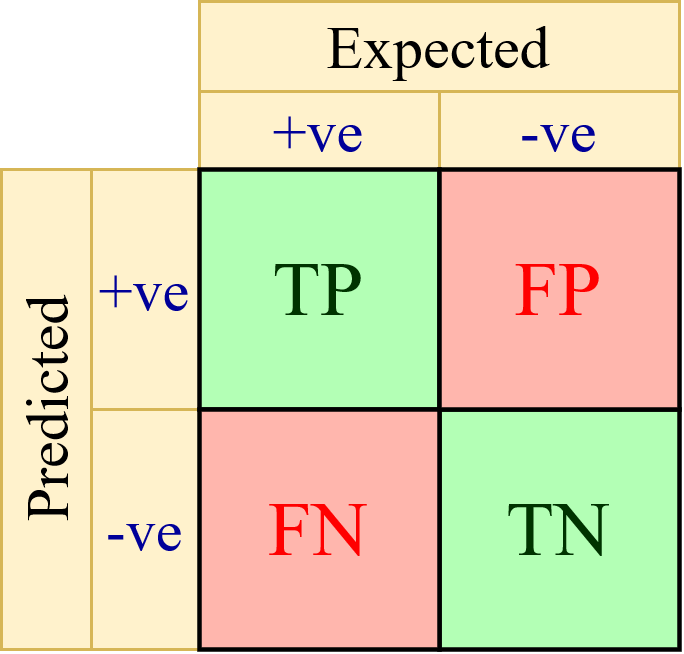Confusion Matrix for a binary-class dataset. Image by the author.

For this binary-class dataset, the precision and recall formulae can be obtained as the following:

Precision = TP/(TP+FP)
Recall = TP/(TP+FN)

Note that precision deals with the Predicted row of the confusion matrix, telling us how accurate the model was in predicting the positive samples out of all the samples predicted to be positive. Recall, on the other hand, deals with the Expected column of the confusion matrix, telling us how accurately the model was able to identify the positive samples out of all positive samples that were actually present.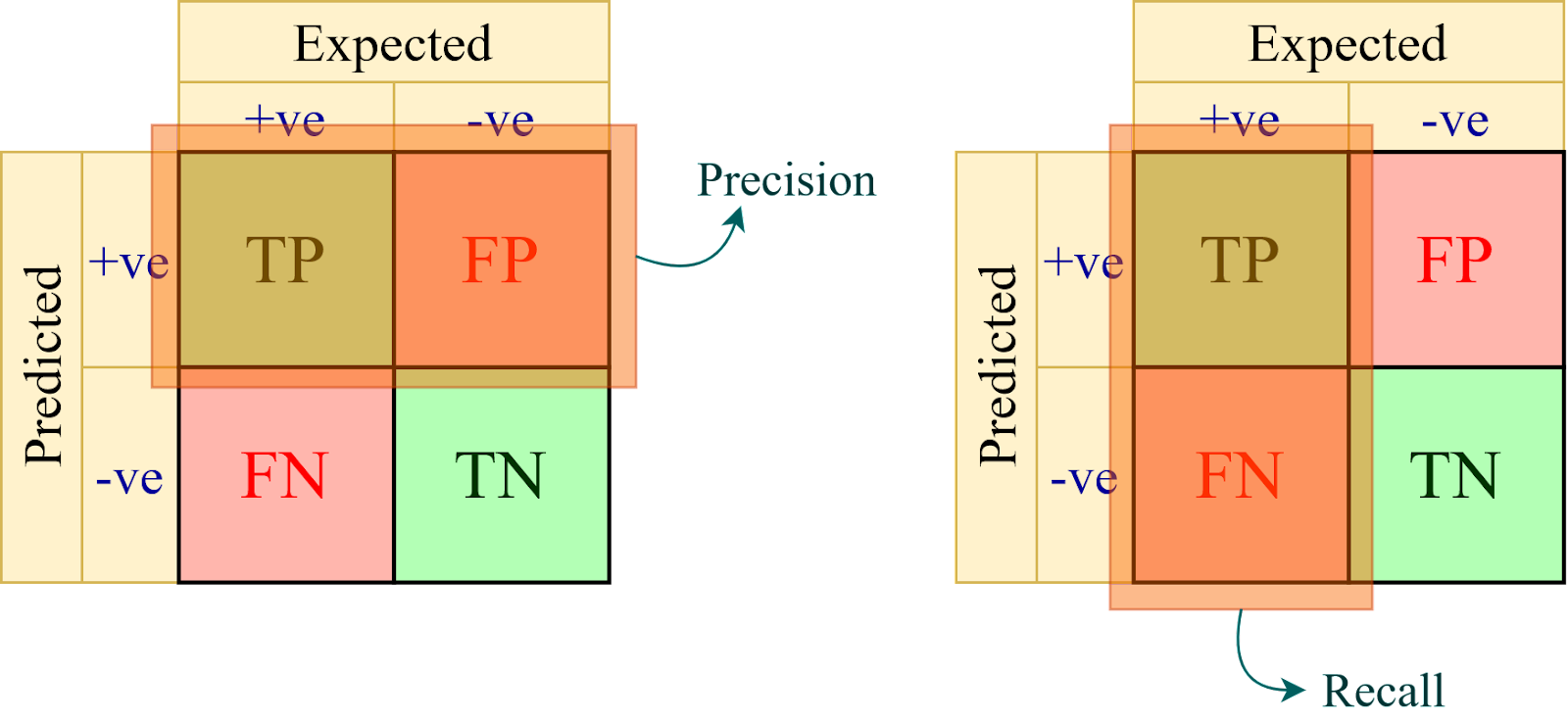Regions of the confusion matrix that help compute precision and recall. Image by the author.

### Precision and Recall for Multi-Class Datasets

The same concept used in the binary-class dataset can be extended to n-classes. The confusion matrix for an n-class dataset is represented as shown below.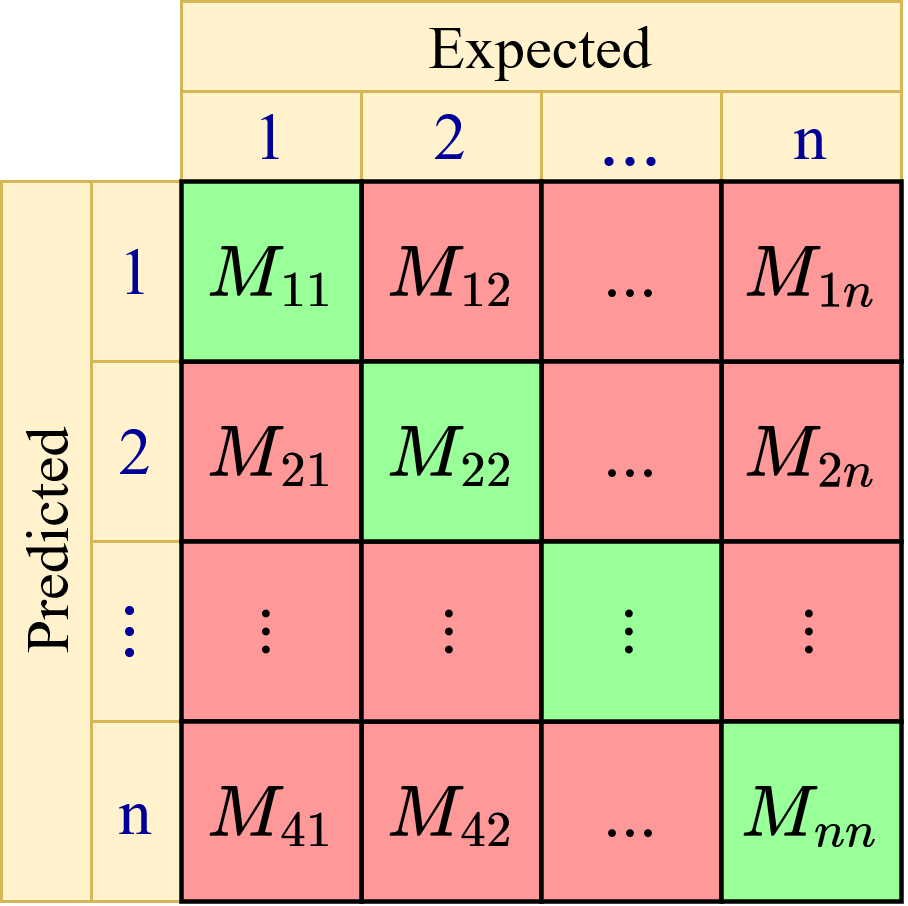Confusion Matrix for an n-class dataset. Image by the author.

The generalized formulae for calculating the class-wise precision and recall metrics are as follows (i = class number):

The class-wise precision and recall scores can then be aggregated using various strategies to compute the global precision and recall values for the whole model. The three main global scores are micro-averaged, macro-averaged, and weighted-averaged precision and recall. Let us look at them next.

#### Micro-Averaged Precision and Recall

The micro-averaged metrics are calculated by considering the net TP, FP, and FN values. For example, the net TP is the sum of the class-wise TP values. Similarly, the net FP and FN values are the sum of the class-wise FP and FN values, respectively. The class-wise TP, FP, and FN values can be easily calculated by converting the multi-class confusion matrix into one-vs-all matrices.

The micro-precision and recall values are calculated as follows:

#### Macro-Averaged Precision and Recall

The macro precision and recall scores are calculated simply by taking the unweighted average of the class-wise precision and recall scores. However, this might not be a reliable indicator in cases where the dataset is imbalanced, i.e., each class of the dataset consists of a different number of samples.

#### Weighted-Averaged Precision and Recall

As the name suggests, the weighted-average metrics are a sample-weighted mean of the class-wise precision and recall values. This is an appropriate global metric system when the dataset is imbalanced.

Where,

## Accuracy, Precision, or Recall—When to Use What

Accuracy is the most commonly used evaluation metric in most data science projects. It tells us how many times our model got its prediction correct as a ratio of the total times the model was used for predictions. However, it makes sense to use a metric like an accuracy only when the dataset is balanced—all classes have the same number of samples. In any case, such a scenario is challenging to realize in practice.

On the other hand, both precision and recall are useful metrics in cases where the dataset is imbalanced (which is valid for almost all practical scenarios). However, depending on the use case, we would like to optimize one or the other metric.

For example, in the case of COVID-19 detection, we want to avoid false negatives as much as possible. COVID-19 spreads easily, and thus we want the patient to take appropriate measures to prevent the spread. A false negative case means that a COVID-positive patient is assessed to not have the disease, which is detrimental. In this use case, false positives (a healthy patient diagnosed as COVID-positive) are not as important as preventing a contagious patient from spreading the disease. In most high-risk disease detection cases (like cancer), recall is a more important evaluation metric than precision.

However, precision is more useful when we want to affirm the correctness of our model. For example, in the case of YouTube recommendations, reducing the number of false positives is of utmost importance. False positives here represent videos that the user does not like, but YouTube is still recommending them. False negatives are of lesser importance here since the YouTube recommendations should only contain videos that the user is more likely to click on. If the user sees recommendations that are not of their liking, they will close the application, which is not what YouTube desires. Most automated marketing campaigns require a high precision value to ensure that a large number of potential customers will interact with their survey or be interested to learn more.

In cases where you want the model to be both precise and sensitive (high recall), computing the F1-score is the way to go. F1-score is the harmonic mean of the precision and recall values, and optimizing the F1-score means optimizing for precision and recall.

## Precision vs Recall

There’s a trade-off between precision and recall, i.e., one comes at the cost of another. Trying to increase precision lowers recall and vice-versa.

With precision, we try to make sure that what we are classifying as the positive class is a positive class sample indeed, which in turn reduces recall. With recall, we are trying not to miss out on any positive class samples, which allows many false positives to creep in, thus reducing precision.

Let’s illustrate this with an example. Suppose we have a binary class dataset where the test set consists of four samples in the “positive” class and six samples in the “negative class.” This is represented as scenario (A) in the diagram below. The RIGHT side of the decision boundary (green line) depicts the positive class, and the LEFT side depicts the negative class.

For this case, precision can be calculated by counting the number of positive class samples on the right side divided by the total number of positive class samples on the right side, which comes out to be 3/5 or 60% in this case. Recall can be calculated by counting the number of positive class samples on the right side divided by the total number of positive class samples, which is 3/4  or 75% in this case.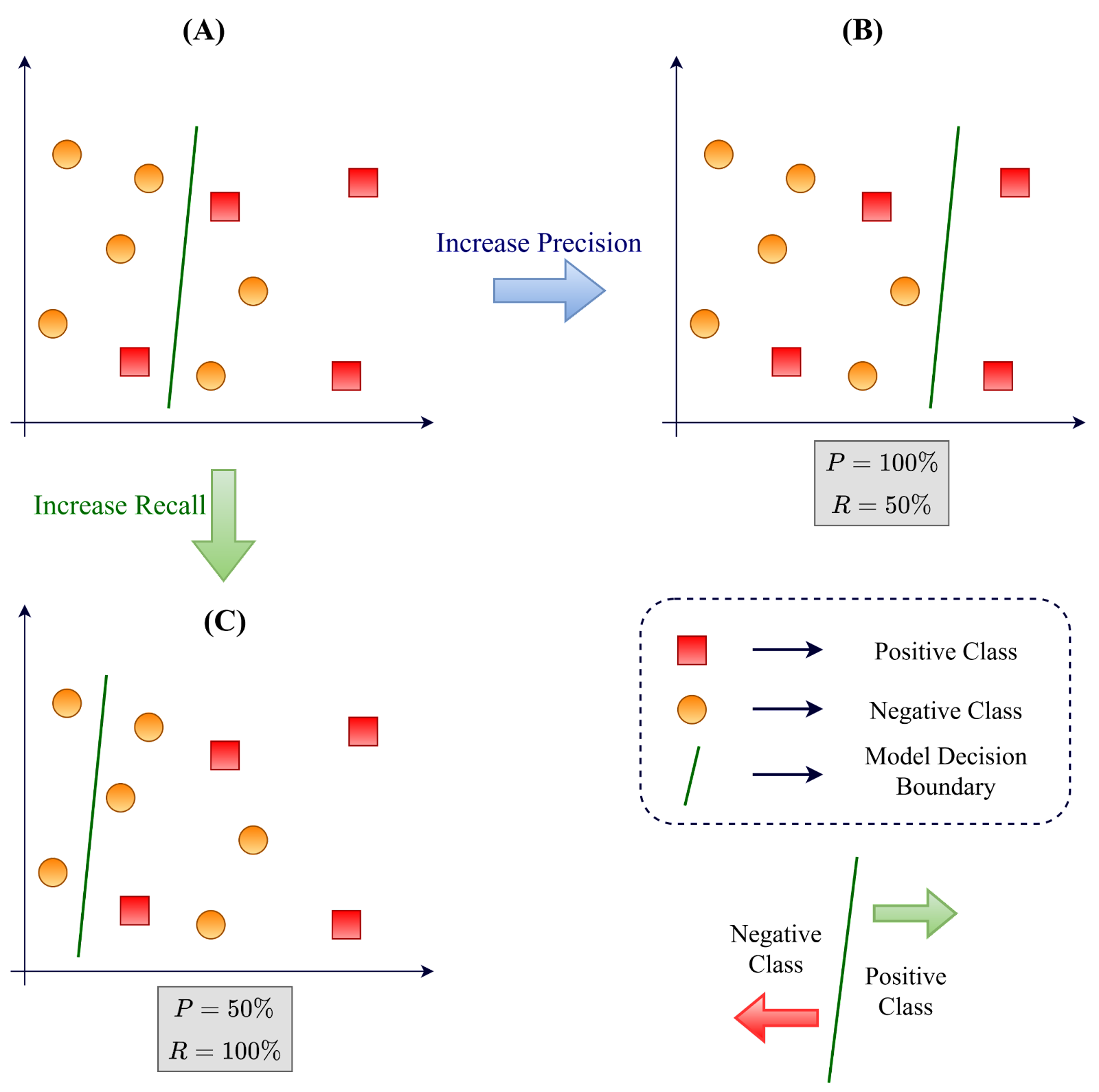Illustration of the Precision-Recall trade-off. Image by the author.

Now, to increase precision, we shift the decision boundary threshold to arrive at scenario (B). Here, precision and recall are:

• Precision = Positive samples on right side/Total samples on right side = 2/2 = 100%
• Recall = Positive samples on right side/Total positive samples = 2/4 = 50%.

Thus, we see that compared to scenario (A), precision increased, but that also resulted in a decreased recall. Here, we tried to scrutinize the positive samples so hard that we missed some of the positive samples while trying to avoid the negative samples from getting to the right side.

From scenario (A), now, if we want to increase the recall score, we arrive at a scenario (C) by changing the decision boundary threshold again. This gives us:

• Precision = Positive samples on right side/Total samples on right side = 4/8 = 50%
• Recall = Positive samples on right side/Total positive samples = 4/4 = 100%

Here, recall jumped to 100%, but at the cost of precision, which is now 50%. In this scenario, while we tried not to miss any of the positive samples, we allowed a lot of negative samples to get on the right side (positive sample side of the decision boundary), leading to a decrease in recall.

### Precision-Recall Curve

Due to the precision-recall trade-off which we discussed above, it is important to track both these metrics while evaluating a model. A precision-recall curve is a plot of precision on the vertical axis and recall on the horizontal axis measured at different threshold values. This curve allows developers to choose the threshold appropriate for their use case.

An example of a precision-recall curve using a randomly generated dataset and logistic regression classifier is shown below. The “No Skill” classifier refers to one that cannot differentiate between different class labels and predicts random classes. For a balanced dataset, the class-wise probabilities will be 50%. It acts as a reference line for the plot of the precision-recall curve.

A perfect classifier (also a reference line in the plot below) can be depicted as the point (1.0, 1.0), that is, both the precision and recall scores are 100%. A practical classifier’s quality can be evaluated based on how much its precision-recall curve bows towards the perfect classifier, i.e., the (1.0, 1.0) point.

The F1-score can be calculated for a specific threshold and thus is the measure of the model’s skill for that threshold. The area under the precision-recall curve (called AUC = Area Under Curve) is a metric that approximates the integral of the precision-recall curve and measures the model’s skill across the entire threshold spectrum.

The AUC for the example curve shown above is 91.9% which is quite good. The AUC of a perfect model is, obviously, 100% because it is simply the area of a square with a side length of 1.0. The AUC of the no-skill model is the area of the rectangle with side lengths 1.0 and 0.0, making AUC = 0%.

## Tools to Compute Precision and Recall

The precision, recall, and AUC metrics can be easily computed in Python using the scikit-learn package. For example, the “precision_score” and the “recall_score” functions take three important arguments (and a few others which we can ignore for now): the expected labels, the true labels, and an “average” parameter which can be binary/micro/macro/weighted/None.

The “binary” mode is for binary class dataset classification only, and it returns the precision or recall metrics using the formula we discussed above. The micro, macro, and weighted modes are for the respective averaging strategies. Furthermore, when the mode is “None,” the function will return a list of class-wise precision or recall scores.

Examples of the usage of the different averaging modes of the precision_score function (on a multi-class dataset) are shown below. The same methods apply to the recall_score function as well.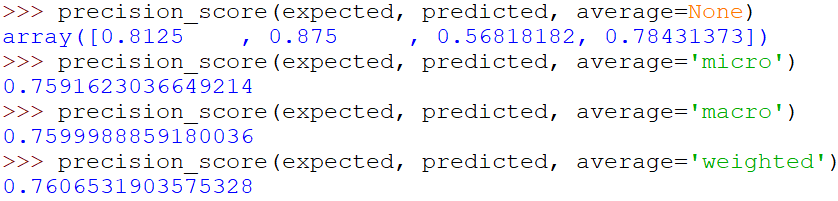Exemplar use of the precision_score function in Python.

If you want all the types of averaging (except “micro”) and the class-wise metrics using one command, using the “classification_report” function of scikit-learn is the way to go. Note that the class-wise, macro, and weighted average metrics obtained above match the “precision” column of the classification report.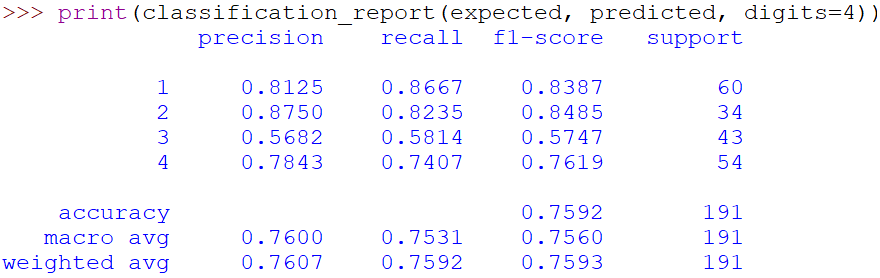Exemplar use of the classification_report function in Python.

Here, “support” refers to the number of samples that were present for computing the metrics. For each class of the data, “support” shows the samples present in that class, and for the global metrics, “support” means the number of samples in the entire test dataset.

For a binary-class dataset, the precision-recall trade-off curve can be plotted in Python using the “precision_recall_curve” function of scikit-learn. However, for that, you need the expected or true labels and the prediction probabilities of the classifier and not the predicted class labels. The function outputs the list of precision, recall, and threshold values. An example of this is shown below.

The precision_recall_curve function outputs the discrete coordinates for the curve. The “matplotlib.pyplot” function of Python is used here to actually plot the curve using the obtained coordinates in a GUI.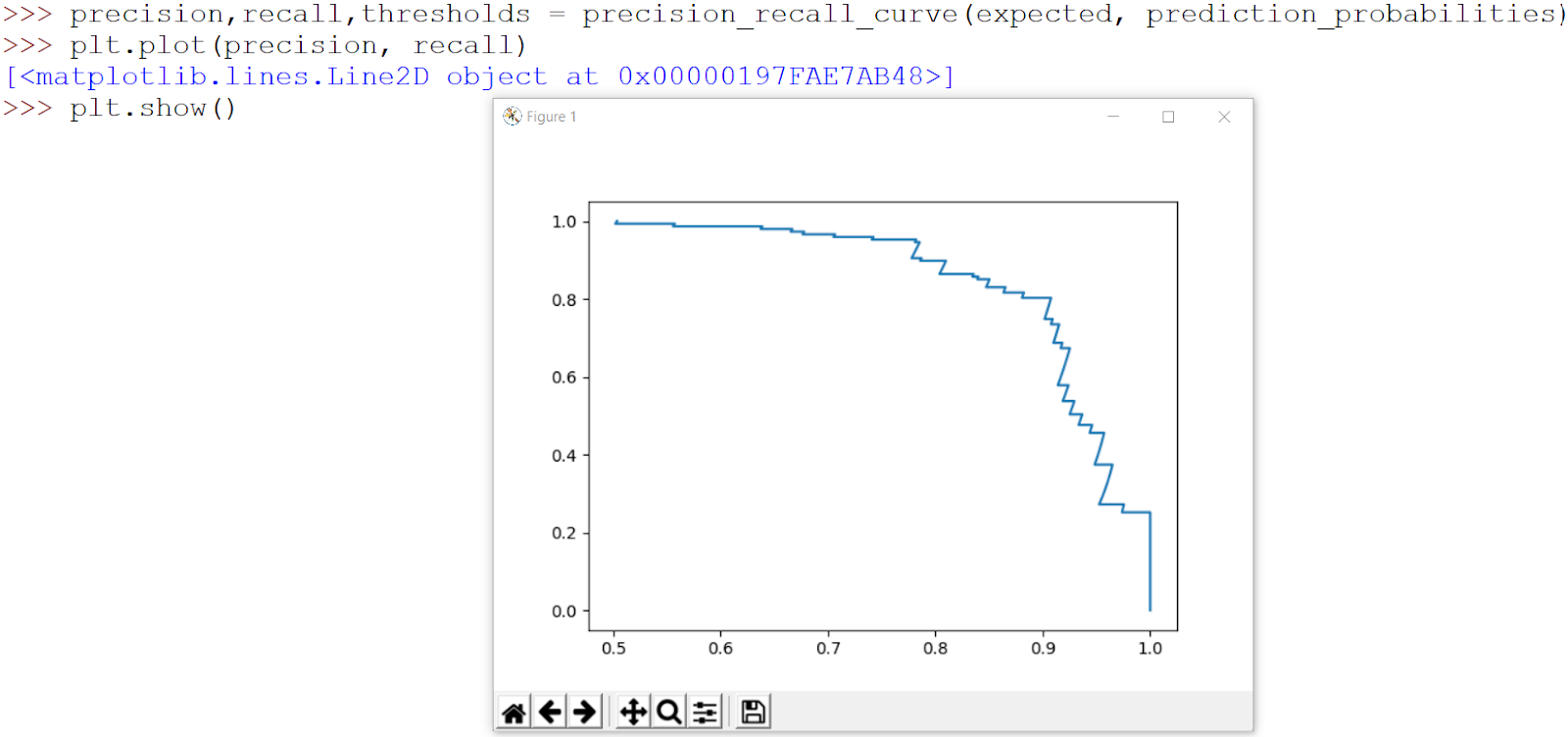Exemplar use of the precision_recall_curve function in Python.

Finally, we can easily calculate the AUC value for the precision-recall curve obtained above using the “auc” function of scikit-learn in Python. Here, we pass the recall and precision value coordinates obtained in the previous function to get the result. An exemplary usage of the function has been shown below.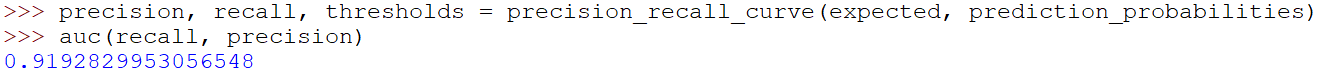Example use of the auc function in Python.

Note that while using the auc function, the recall score needs to be the first argument, and the precision score is the second argument. While plotting the precision-recall curve, we had the recall in the horizontal axis (or x-axis) and precision in the vertical axis (or y-axis), so the x-component or independent axis needs to be the first argument. The auc function computes the curve and calculates the integral value on its own.

## Key Takeaways

Knowing how to evaluate a machine learning model fairly is as important as knowing how to train a model. While accuracy is the most popularly used metric, it is not a comprehensive evaluation scheme for a classifier model. Precision and recall are metrics that help identify knowledge gaps in the model through class-wise evaluations.

However, precision and recall need to be optimized differently based on the application of the model. Knowing when to use which metric is essential for an ML engineer. Global versions of the precision and recall metrics can also be calculated using different averaging strategies, which we have discussed in detail.

Precision and recall offer a trade-off based on the decision thresholds, which can be visualized from the precision-recall curve. A good classifier tries to maximize both metrics, thus increasing the area under the curve value, which represents a classifier’s quality across all thresholds.

Computation of the class-wise and global precision and recall metrics, plotting the precision-recall curve, and calculating AUC and F1-score, are easily achieved using only a couple of lines of simple code in Python. Comprehensively looking at these metrics to make decisions for model updates allows the classifier to be tailored for optimal performance in its application.

FREE
Apply for an Education Plan
Thank you! Your submission has been received!
Oops! Something went wrong while submitting the form.

## Related articles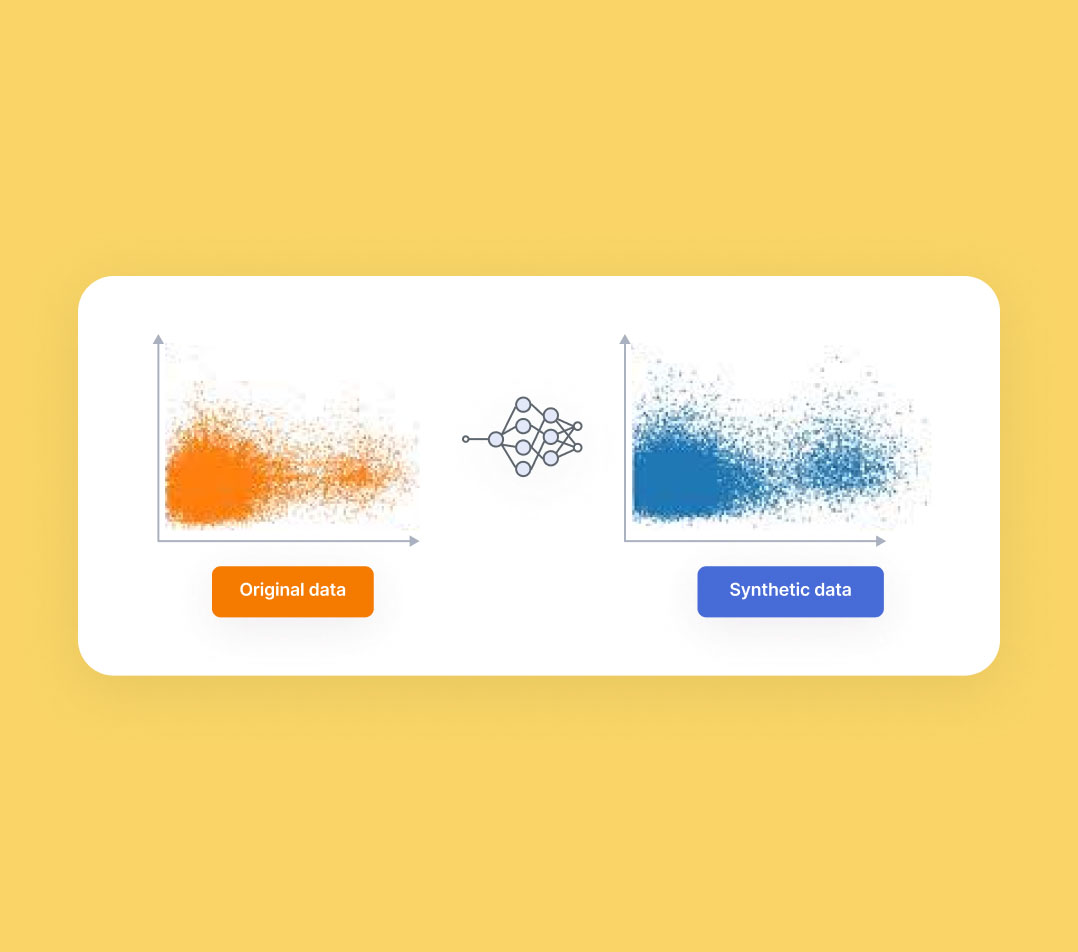What is Synthetic Data in Machine Learning and How to Generate ItThe Essential Guide to Zero-Shot Learning 
Subscribe to our blog
1 personalized email from V7's CEO per month
Thank you for subscribing!
Oops! Something went wrong while submitting the form.